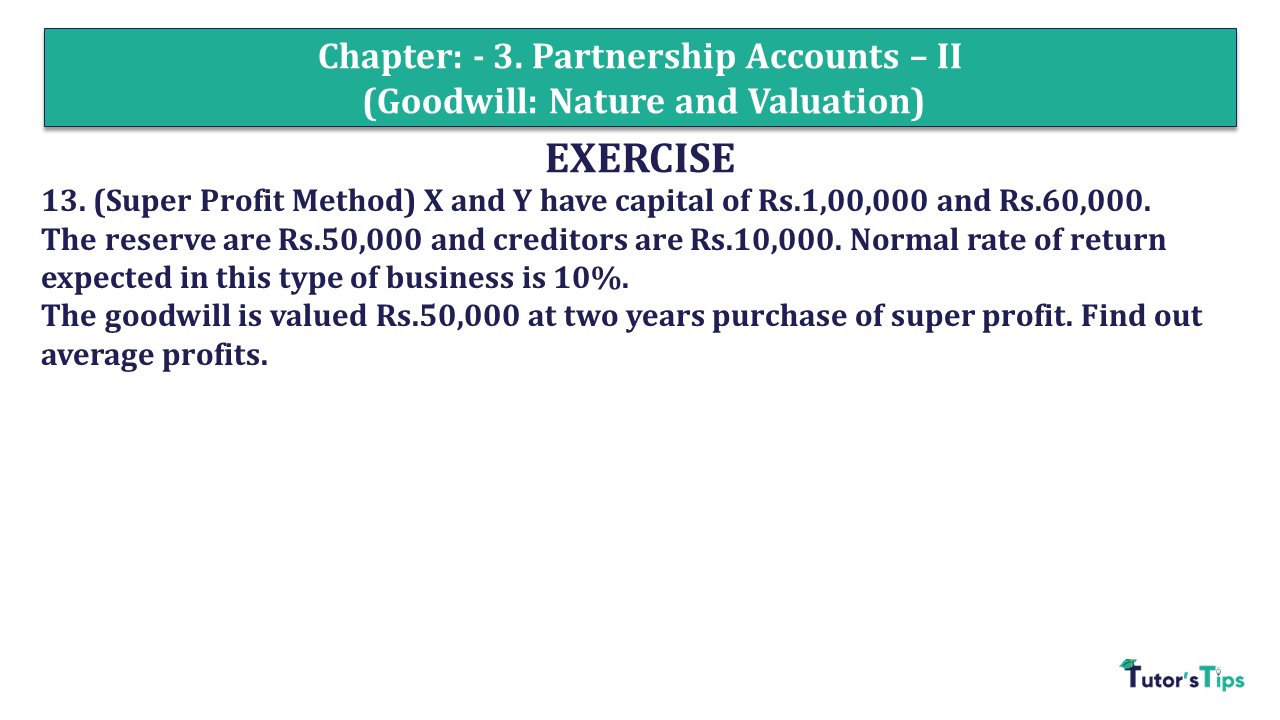# Question 13 Chapter 3 of +2 Part-1 – USHA Publication 12 Class Part – 1Q-13 - CH-3 - Usha +2 Book 2018 - Solution

Question 13 Chapter 3 of +2-Part-1

13. (Super Profit Method) X and Y have capital of Rs.1,00,000 and Rs.60,000.
The reserve are Rs.50,000 and creditors are Rs.10,000. Normal rate of return expected in this type of business is 10%.
The goodwill is valued Rs.50,000 at two years purchase of super profit. Find out average profits.

### The solution of Question 13 Chapter 3 of +2 Part-1: –

 Capital Employed = 1,00,000 + 60,000 + 50,000 = 2,10,000

 Normal Profit = Capital Employed X Normal Rate of Return 100 = 2,10,000 X 10 100 = 21,000 Goodwill of Firm = 50,000(Given)

 Super Profit = Firm Goodwill Number of years = 50,000 2 = 25,000

 Average Profit = Normal Profit + Super Profit = 21,000 + 25,000 = 46,000

Comment if you have any questions.

Also, Check out the solved question of previous Chapters: –